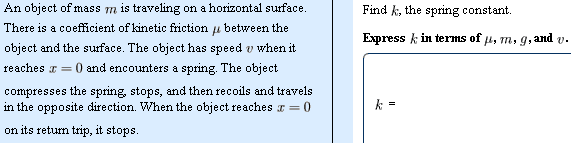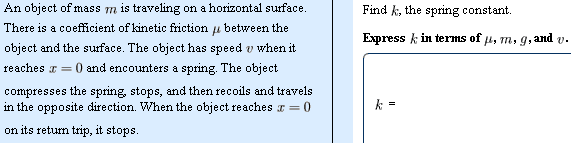# Potential energy and energy conservation problem

## Homework Statement## Homework Equations

K$$_{1}$$+U$$_{1}$$ +W$$_{nc}$$ =K$$_{2}$$ + U $$_{2}$$ (The subscript coding is not working right, but they ARE suppose to be subscripts and nothing is squared)

Work on the spring = .5kx$$_{2}$$ - .2kx$$_{2}$$ (final minus initial)

## The Attempt at a Solution

I didnt use the 1st equation because this problem doesn't involve any up and down movements (y direction / same potential all over).

So I tried the 2nd but because to net work is 0 both x (distance) become 0 also and I can't have any way of solving for k. They don't tell us how much it compressed so I can't make a new final / initial point.

What am I missing?

HallsofIvy
Science Advisor
Homework Helper

## Homework Statement## Homework Equations

K$$_{1}$$+U$$_{1}$$ +W$$_{nc}$$ =K$$_{2}$$ + U $$_{2}$$ (The subscript coding is not working right, but they ARE suppose to be subscripts and nothing is squared)
It is much better (and easier) to put an entire equation in LaTex, not parts of it. Here, you subscripts look like superscripts because the "bases" of the standard text and LaTex are different. Much better is
$$K_1+ U_1+ W_{nc}= K_2+ U_2$$

Work on the spring = .5kx$$_{2}$$ - .2kx$$_{2}$$ (final minus initial)
What is x here?

## The Attempt at a Solution

I didnt use the 1st equation because this problem doesn't involve any up and down movements (y direction / same potential all over).

So I tried the 2nd but because to net work is 0 both x (distance) become 0 also and I can't have any way of solving for k. They don't tell us how much it compressed so I can't make a new final / initial point.

What am I missing?
It's hard to tell because you haven't said what the "x" in your formula represents. Also the friction force is equal to the coefficient, $\mu$, times the speed isn't it? If x is the greatest compression of the spring, then the work done by friction, which is the integral of kv(t), plus the work done by the spring to stop the object must equal the kinetic energy of the object initially. Of course, you get the work from the spring back again during the "recoil". If the object stops at x= 0, then the loss of energy to friction must be equal to the initial kinetic energy of the object. That will depend upon the distance required for the greatest compression of the spring which, in turn, tells you k.

It's hard to tell because you haven't said what the "x" in your formula represents. Also the friction force is equal to the coefficient, $\mu$, times the speed isn't it? If x is the greatest compression of the spring, then the work done by friction, which is the integral of kv(t), plus the work done by the spring to stop the object must equal the kinetic energy of the object initially. Of course, you get the work from the spring back again during the "recoil". If the object stops at x= 0, then the loss of energy to friction must be equal to the initial kinetic energy of the object. That will depend upon the distance required for the greatest compression of the spring which, in turn, tells you k.

Sorry, X is the distance compressed on the spring
and I thought the friction force is equal to the $\mu$ times the normal force?

HallsofIvy
Science Advisor
Homework Helper
Okay, that's one kind of friction force. If that's what you were given, then that's what you should use.

Initially, the object has speed v and so kinetic energy (1/2)mv2. In compressing the spring a distance X, the object will have increased the potential energy of the spring (1/2)kX2 (integral of kx from 0 to X). The friction force is mg$\mu$ and so in distance X will have done work mg$\mu$X on the object. If it comes to a halt in that distance, all of the object's kinetic energy must have gone into the potential energy of the spring or lost to friction: (1/2)kx2+ mg$\mu$X= (1/2)mv2. That's a quadratic equation for X.

Any energy lost in compressing the spring is regained when it extends again. The energy lost to friction, however is the same moving in both directions so the total energy lost= total energy lost to friction= 2(mg$\mu$X. If the object stops at the same position at which it initially met the spring, that must be equal to the initial kinetic energy: 2mg$\mu$X= (1/2)mv2. Since X will depend upon k, you can solve that for k.

What equation did you use to get (1/2)kx2+ mg$\mu$X= (1/2)mv2

I understand how you got the (1/2)kx2 (because you took the sum of each force during the spring compression) and I also under the kinetic energy but what equation did you use to put them together like that

HallsofIvy
Science Advisor
Homework Helper
"Conservation of Energy", of course. The right side is the total energy of the object just as it meets the spring (kinetic energy only since it has not yet started to compress the spring). The left side is the total energy of the object at maximum spring compression (potential energy only since now the velocity is 0) plus energy lost to friction.

"Conservation of Energy", of course. The right side is the total energy of the object just as it meets the spring (kinetic energy only since it has not yet started to compress the spring). The left side is the total energy of the object at maximum spring compression (potential energy only since now the velocity is 0) plus energy lost to friction.

So just to clarify
You used the $$K_{1}$$+$$U_{1}$$+$$W_{nc}$$=$$K_{2}$$+$$U_{2}$$

Except in this case because it is not gravatational potential it is elastic so the U (potential energy) in this case is 1/2k$$x^{2}$$ right?
And $$W_{nc}$$ is the total non conservative forces such as friction?

If that is the case, why did you say the right side is the total energy of the object just as it meets the spring when in the problem that was the initial starting point (in the equation the right side is the 2nd point)? Are we allowed to change the points freely?

is that right?

HallsofIvy
Science Advisor
Homework Helper
"Conservation of energy" means that the total energy- potential energy, kinetic energy and energy lost to friction, is the same at any two points.

Ok so what you just said made sense. So next I tried to do the problem again by choosing my points at when it first touch the spring and just before it decompresses (like what you had set up), my following equation I had this

using $$K_{1}$$+$$U_{1}$$+$$W_{nc}$$=$$K_{2}$$+$$U_{2}$$

1/2m$$v^{2}$$ + 0 + xmg$$\mu$$ = 0 + 1/2k$$x^{2}$$

On the left side
When it hits the spring there is 0 potential because it has not yet compress the spring and it still remains on a horizonal surface. It has a kinetic energy and friction force
On the right side
I have 0 kinetic with potential energy.

So solving for K i get

$$\frac{mv^{2}+xmg\mu}{x^{2}}$$

But according to the answer it does not depend on the variable X (distance compressed by spring)

Last edited: